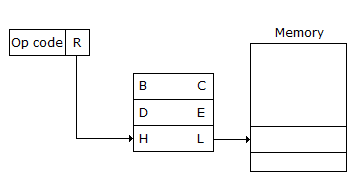# Electronics and Communication Engineering - Microprocessors

### Exercise :: Microprocessors - Section 6

6.

Assertion (A): In C it is possible for functions to call themselves.

Reason (R): C makes use of 'Pointers'.

 A. Both A and R are correct and R is correct explanation of A B. Both A and R are correct but R is not correct explanation of A C. A is correct R is wrong D. A is wrong R is correct

Explanation:

No answer description available for this question. Let us discuss.

7.

In IC 74181 a sum greater than 15 leads to low carry out.

 A. True B. False

Explanation:

No answer description available for this question. Let us discuss.

8.

Assertion (A): In 8085, the zero flag is set when ALU operation results in 0. If the result is not 0, this flag is reset.

Reason (R): In 8085 the S flag is set when the contents of accumulator become negative during execution of an instruction.

 A. Both A and R are correct and R is correct explanation of A B. Both A and R are correct but R is not correct explanation of A C. A is correct R is wrong D. A is wrong R is correct

Explanation:

No answer description available for this question. Let us discuss.

9.

The operations which occur most frequently in a computer are

 A. fetch B. execute C. both (a) and (b) D. none of the above

Explanation:

No answer description available for this question. Let us discuss.

10.

The addressing mode depicted in the given figure isA. direct B. register C. register indirect D. immediate